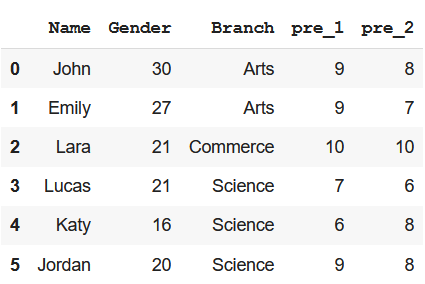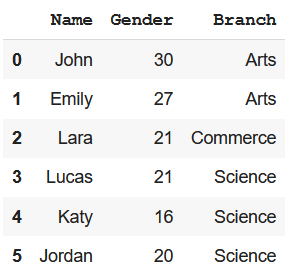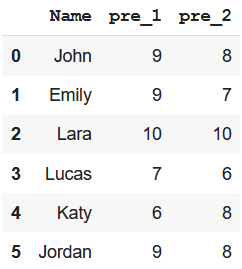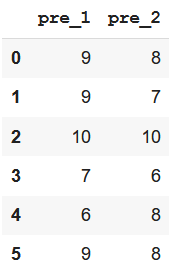Open in App
Not now

# Python – Subset DataFrame by Column Name

• Last Updated : 16 Mar, 2021

Using Pandas library, we can perform multiple operations on a DataFrame. We can even create and access the subset of a DataFrame in multiple formats. The task here is to create a subset DataFrame by column name. We can choose different methods to perform this task. Here are possible methods mentioned below –

Before performing any action, we need to write few lines of code to import necessary libraries and create a DataFrame.

Creating the DataFrame

## Python3

 `#import pandas``import` `pandas as pd`` ` `# create dataframe``data ``=` `{``'Name'``: [``'John'``, ``'Emily'``, ``'Lara'``, ``'Lucas'``, ``'Katy'``, ``'Jordan'``], ``        ``'Gender'``: [``30``, ``27``, ``21``, ``21``, ``16``, ``20``], ``        ``'Branch'``: [``'Arts'``, ``'Arts'``, ``'Commerce'``, ``'Science'``, ``                   ``'Science'``, ``'Science'``],``        ``'pre_1'``: [``9``, ``9``, ``10``, ``7``, ``6``, ``9``],``        ``'pre_2'``: [``8``, ``7``, ``10``, ``6``, ``8``, ``8``]}`` ` `df ``=` `pd.DataFrame(data)``df`

Output:Method 1: Using Python iloc() function

This function allows us to create a subset by choosing specific values from columns based on indexes.

Syntax:

`df_name.iloc[beg_index:end_index+1,beg_index:end_index+1]`

Example: Create a subset with Name, Gender and Branch column

## Python3

 `# create a subset of all rows``# and Name, Gender and Branch column``df.iloc[:, ``0``:``3``]`

Output :Method 2: Using Indexing Operator

We can use the indexing operator i.e. square brackets to create a subset dataframe

Example: Create a subset with Name, pre_1, and pre_2 column

## Python3

 `# creating subset dataframe using ``# indexing operator``df[[``'Name'``, ``'pre_1'``, ``'pre_2'``]]`

Output –Method 3: Using filter() method with like keyword

We can use this method particularly when we have to create a subset dataframe with columns having similarly patterned names.

Example: Create a subset with pre_1 and pre_2 column

## Python3

 `# create a subset of columns pre_1 and pre_2``# using filter() method``df.``filter``(like``=``'pre'``)`

Output:My Personal Notes arrow_drop_up